Ex 3.4

Chapter 3 Class 10 Pair of Linear Equations in Two Variables
Serial order wise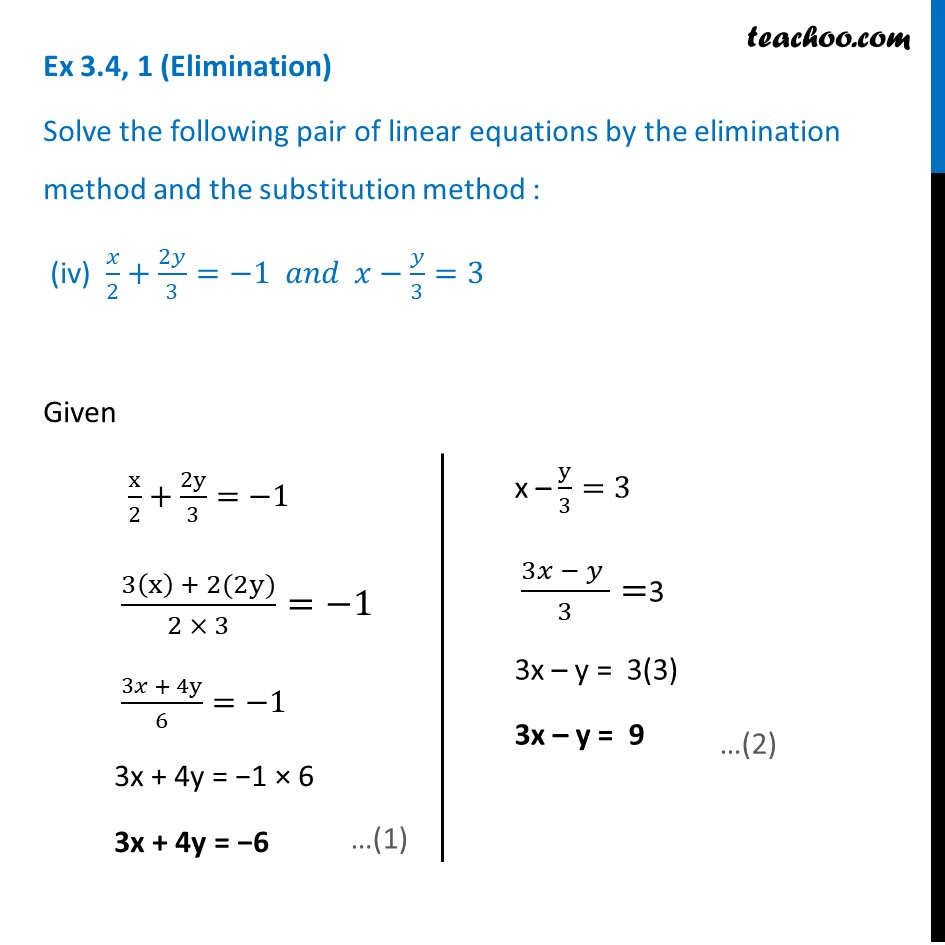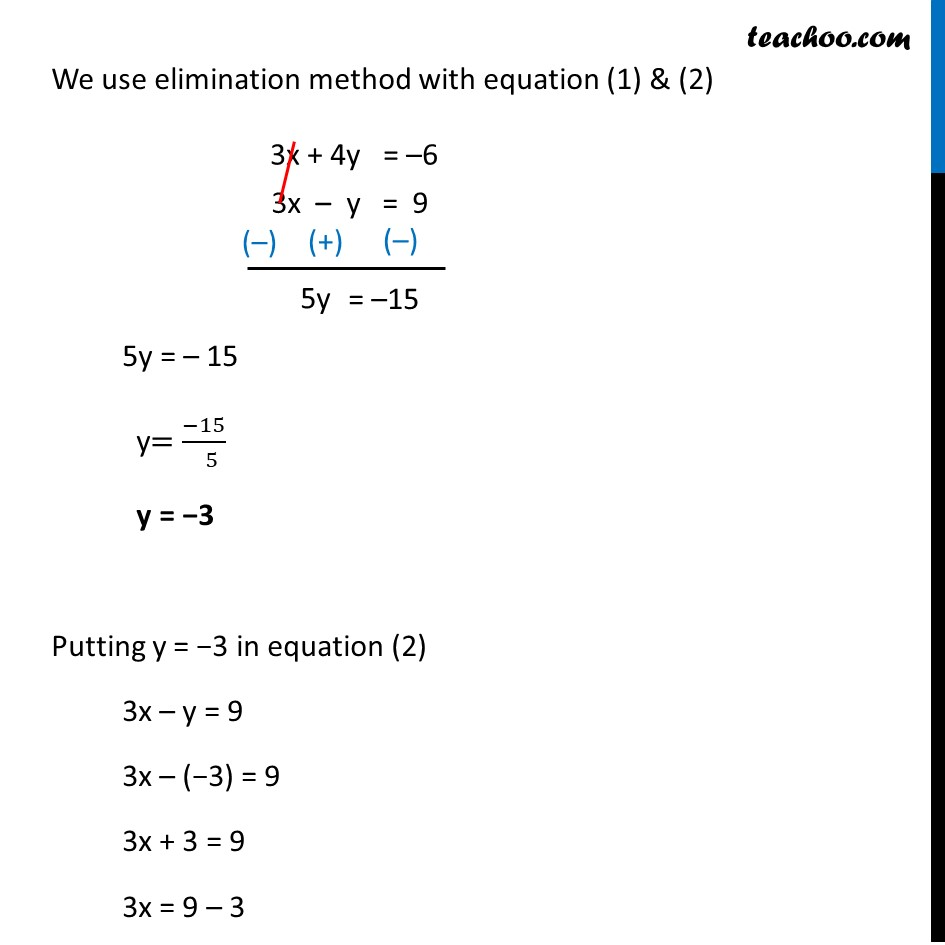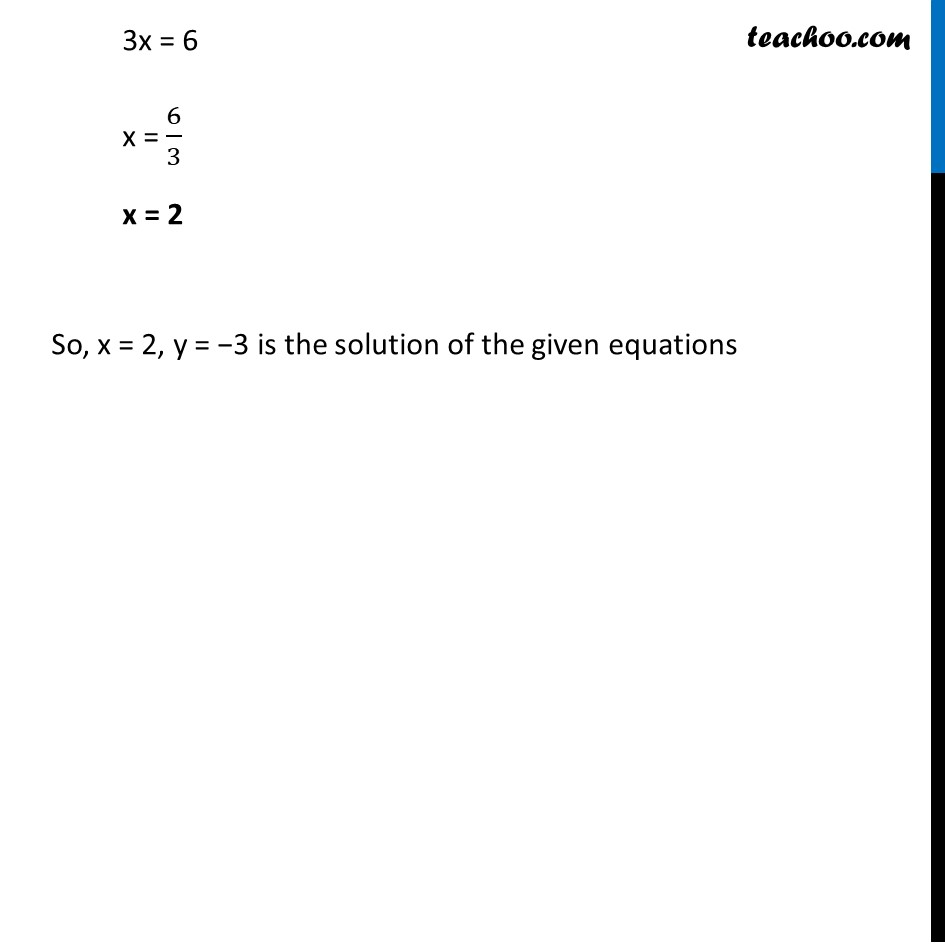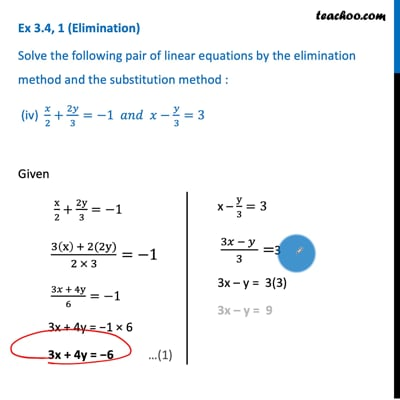This video is only available for Teachoo black users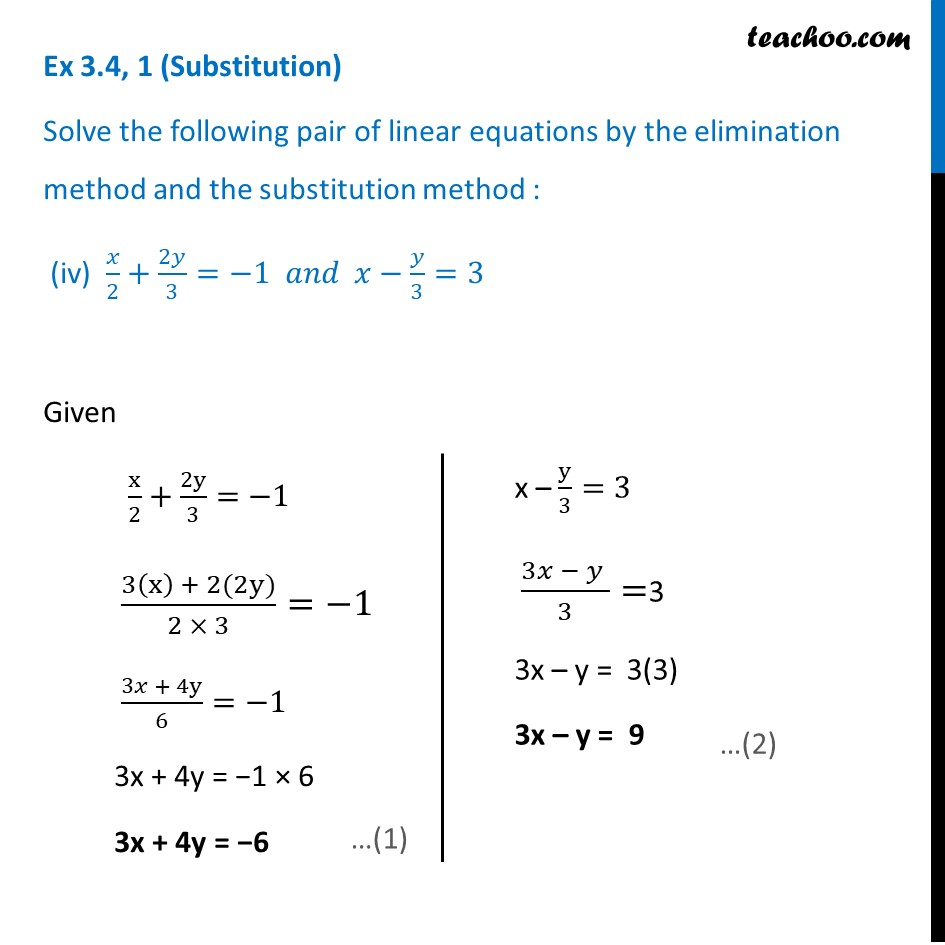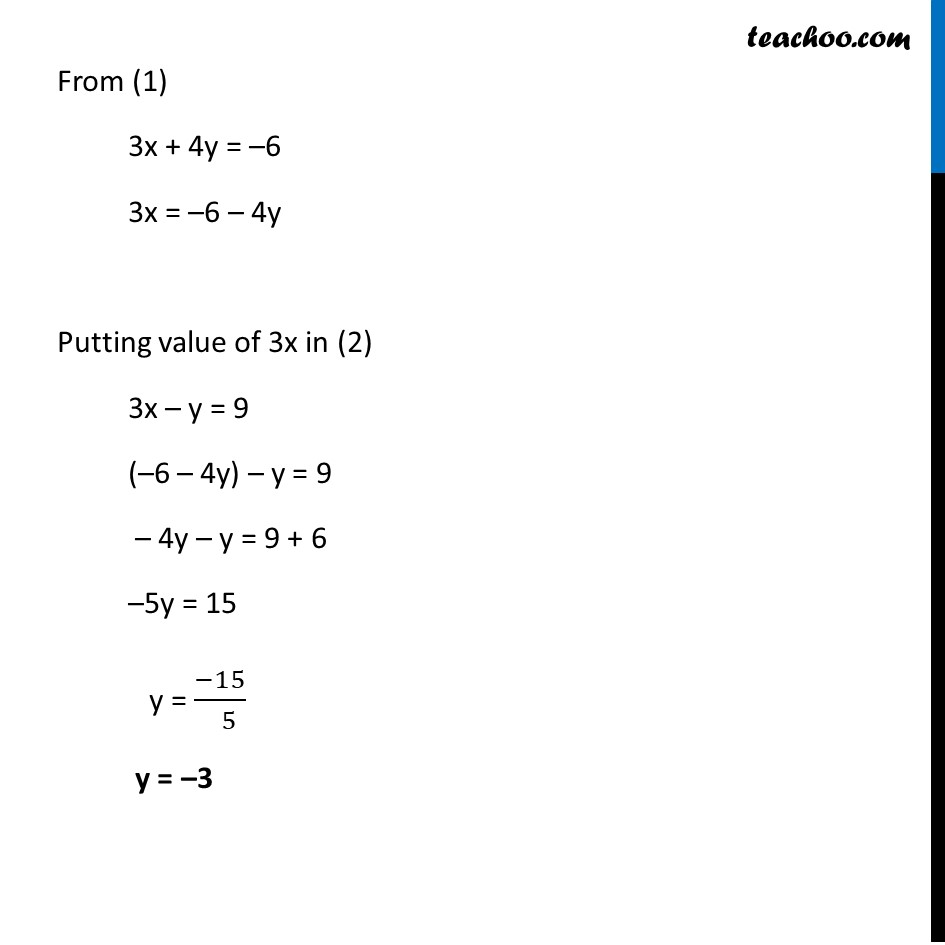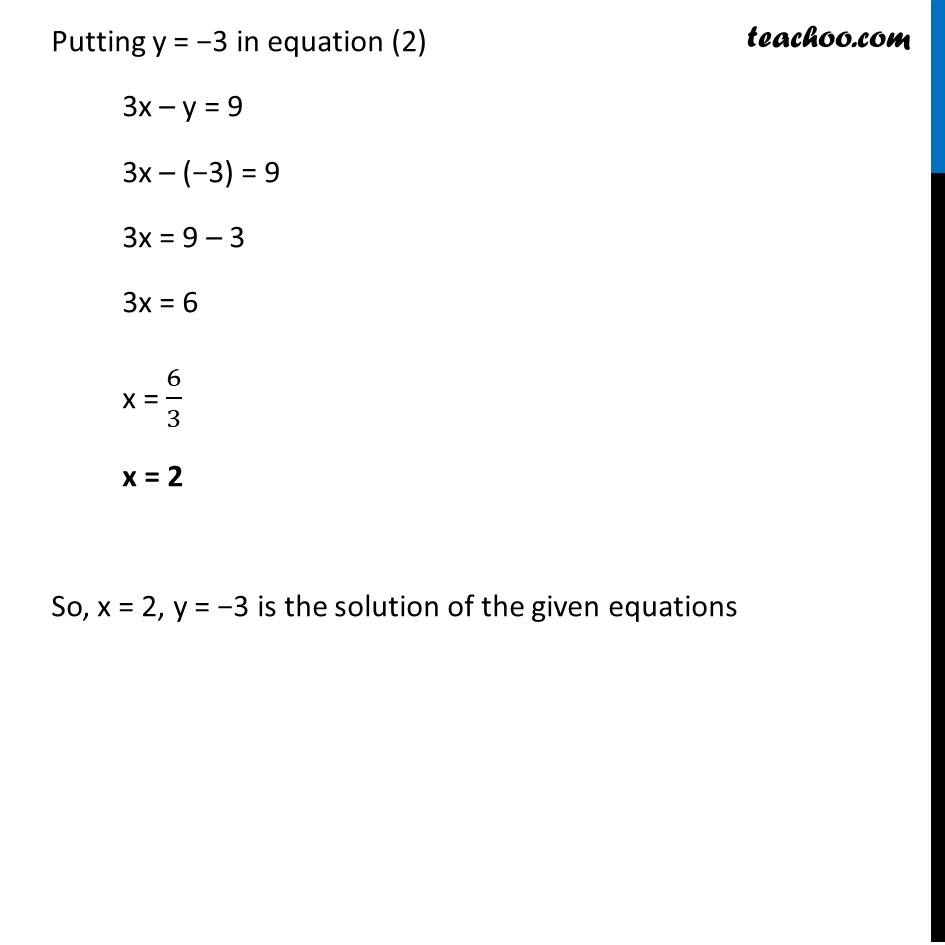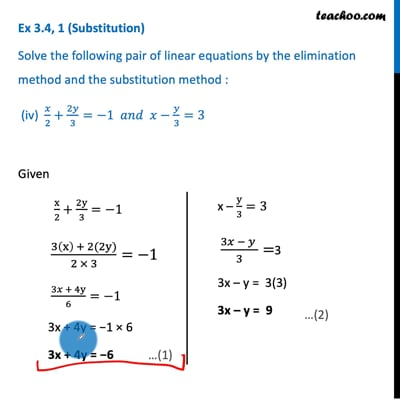This video is only available for Teachoo black users

Introducing your new favourite teacher - Teachoo Black, at only ₹83 per month

### Transcript

Ex 3.4, 1 (Elimination) Solve the following pair of linear equations by the elimination method and the substitution method : (iv) 𝑥/2+2𝑦/3=−1 𝑎𝑛𝑑 𝑥−𝑦/3=3 Given x/2+2y/3=−1 (3(x) + 2(2y))/(2 × 3)=−1 (3𝑥 + 4y)/6=−1 3x + 4y = −1 × 6 3x + 4y = −6 x – y/3=3 (3𝑥 − 𝑦 )/3=3 3x – y = 3(3) 3x – y = 9 We use elimination method with equation (1) & (2) 5y = – 15 y=(−15)/( 5) y = −3 Putting y = −3 in equation (2) 3x – y = 9 3x – (−3) = 9 3x + 3 = 9 3x = 9 – 3 3x = 6 x = 6/3 x = 2 So, x = 2, y = −3 is the solution of the given equations Ex 3.4, 1 (Substitution) Solve the following pair of linear equations by the elimination method and the substitution method : (iv) 𝑥/2+2𝑦/3=−1 𝑎𝑛𝑑 𝑥−𝑦/3=3 Given x/2+2y/3=−1 (3(x) + 2(2y))/(2 × 3)=−1 (3𝑥 + 4y)/6=−1 3x + 4y = −1 × 6 3x + 4y = −6 x – y/3=3 (3𝑥 − 𝑦 )/3=3 3x – y = 3(3) 3x – y = 9 From (1) 3x + 4y = –6 3x = –6 – 4y Putting value of 3x in (2) 3x – y = 9 (–6 – 4y) – y = 9 – 4y – y = 9 + 6 –5y = 15 y = (−15)/( 5) y = –3 Putting y = −3 in equation (2) 3x – y = 9 3x – (−3) = 9 3x = 9 – 3 3x = 6 x = 6/3 x = 2 So, x = 2, y = −3 is the solution of the given equations